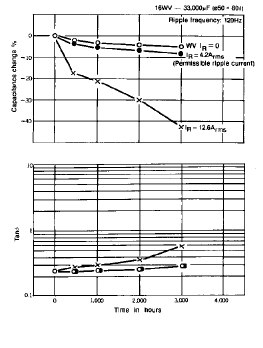The life of aluminum electrolytic capacitors is very dependent on environmental and electrical factors. Environmental factors include temperature, humidity, atmospheric pressure and vibration. Electrical factors include operating voltage, ripple current and charge-discharge duty cycle. Among these factors, temperature (ambient temperature and internal heating due to ripple current) is the most critical to the life of aluminum electrolytic capacitors. Whereas, conditions such as vibration, shock and humidity have little affect on the actual life of the capacitor.

Aluminum electrolytic capacitors are evaluated by accelerated life tests. The acceleration tests contain three factors (one for temperature, voltage and ripple current) which are shown by the following equation:

LB = LA * AT * AV * AR

Where:
LB = Lifetime under a certain condition “B”
LA = Lifetime under a certain condition “A”
AT = Temperature acceleration factor
AV = Voltage acceleration factor
AR = Ripple current acceleration factor

Effects of Temperature on Life

Because a capacitor is essentially an electrochemical device, increased temperatures accelerate the chem ical reaction rates within the capacitor (usually a 10°C rise in temperature will double the chemical reaction rate). Therefore, higher temperatures cause accelerated changes in decreasing capacitance and increasing tan d due to the gradual evaporation of the electrolyte through the capacitor seal (see Figure 2). The Equivalent Series Resistance change (DESR) can be a measure of electrolyte loss and has been found experimentally to be dependent on temperature as shown in Figure 3. In this figure, the vertical axis is the ratio of DESR at some particular temperature to the normalized ESR at a reference temperature (60° or 85°C for these examples). The DESR at both temperatures is taken over the same test time.

Figure 2. Load Life Dependence on Temperature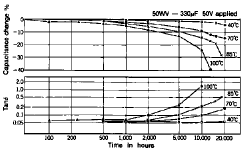Figure 3. Accelerated Temperature Test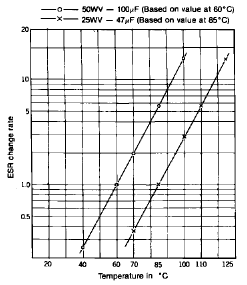The temperature acceleration factor and its relationship with capacitance change, ESR and electrolyte loss are shown below.

(1) The relationship between Delta Cap and ESR versus weight loss of the electrolyte (Delta W) at various temperatures versus time. As might be expected, the degradation of capacitor characteristics occurs more rapidly at higher temperatures as shown in Figure 4. Also note that Delta Cap increases proportionally to the loss of electrolyte while ESR increases in a more rapid fashion.

Figure 4. The Relationship Between Ambient Temperature and Delta Cap, ESR and Delta W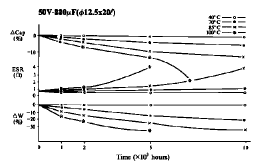Figure 5. Electrolyte Loss on Life Test versus Temperature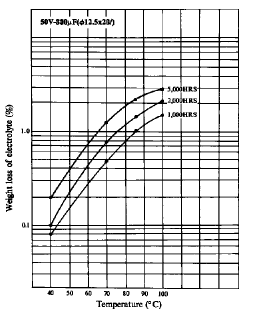(2) The relationship between the temperature acceleration factor and the weight loss of electrolyte. Figure 5 shows the results of a typical test in which the weight loss of the electrolyte increased 1.9 times with a temperature rise of 10°C. The ESR shows a similar change due to a rise in temperature. Generally the temperature acceleration factor is between 1.7 to 2.3, depending upon the particular samples. From these results we can formulate the equation:Where:
Delta T = the “acceleration test temperature” minus the “actual operating temperature”
AT = the acceleration factor.

Figure 6 shows the acceleration factor at different operating temperatures for several capacitor ratings, relative to performing at 85°C.

Figure 6. Temperature Acceleration Factor Calculated by ESR Change.
(The factor at 85°C is defined as 1.)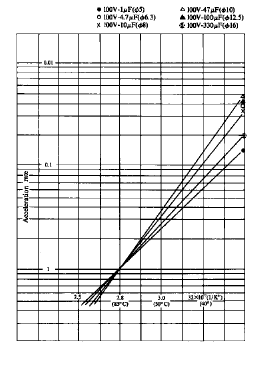Effects of Voltage on Life

Voltage within the allowed operating range has little effect on the actual life expectancy of a capacitor. However in certain applications or misapplications, the applied voltage can be detrimental to the life of an aluminum electrolytic capacitor.

(a) Operating Voltage

When in service at voltages equal to or below the rated value, the life of electrolytic capacitors is affected less by applied voltage than by operating temperature. Figures 7, 8 and 9 show life test results with various reduced voltages applied. The curves show that the life of the capacitor has not been significantly increased by a reduction in voltage. This is due to the use of proper forming voltages to minimize gas generation and leakage current. From this we can say that when capacitors are used at or below their rated voltage, the acceleration factor AV is equal to 1.

Figure 7. Applied Voltage and Life Expectancy at 85°C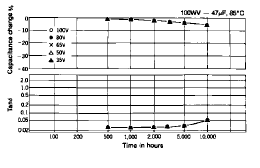Figure 8. Applied Voltage and Life Expectancy at 105°C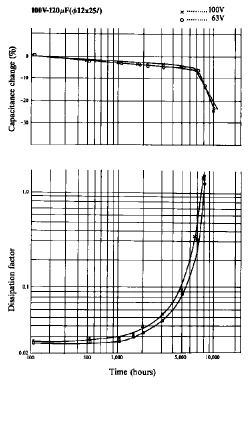Figure 9. Applied Voltage and Life Expectancy at 85°C(b) Reverse Voltage

The application of reverse voltage causes internal heating and oxidation of the cathode foil, thus generating gas as shown in the following formula:

2Al + 3H2O – 6e -> Al2O3 + 6H+
6H+ + 6e -> 3H2^

This pressure build up may cause the safety vent to open or possibly destroy the capacitor. Deterioration is slow with a reverse voltage of a few volts. (See Figure 10.)

(c) Excessive Voltage

Continuous application of excessive operating voltages will rapidly increase the leakage current. (See Figures 11 and 12.) Internal heating and gas generation caused by increased leakage current may destroy the capacitor.

Figure 10. Reverse Voltage at 85°C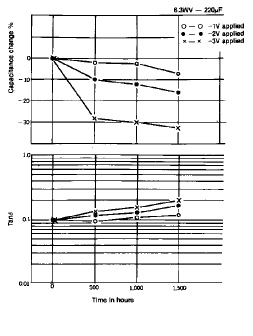Figure 11. Voltage and IDCL Characteristics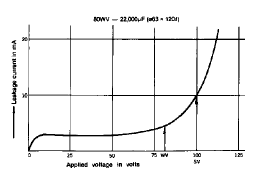Figure 12. Excessive Operating Voltage at 85°C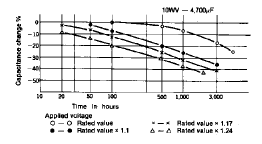(d) Charge-Discharge Duty

When designing heavy duty charge-discharge circuits, customers are recommended to select capacitors specially designed for this application. Graphs showing the characteristics of these capacitors under typical charge-discharge applications are shown in Figures 13-15.

Figure 13. Heavy Charge-Discharge Duty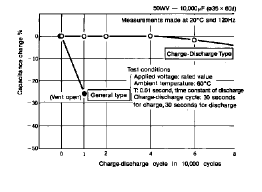Figure 14. Heavy Charge-Discharge Duty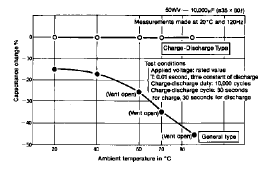General purpose type aluminum electrolytic capacitors will show rapid capacitance drops caused by oxidation of the cathode foil by discharge currents. Furthermore, discharge currents may cause heat rise and pressure increases resulting in venting and the potential destruction of the capacitor.

The rate of deterioration during heavy charge-discharge duty is dependent on temperature and applied voltage. (See Figures 14 and 15.)

Figure 15. Heavy Charge-Discharge Duty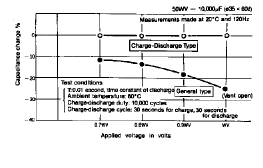Effects of Ripple Current on Life

Compared with other types of capacitors, aluminum electrolytic capacitors have higher tan d and therefore are subject to greater internal heat generation when ripple currents exist. To assure the capacitor’s life, the maximum permissible ripple current of the product is specified.

When ripple current flows through the capacitor, heat is generated by the power dissipated in the capacitor accompanied by a temperature increase. Internal heating produced by ripple currents can be represented by:

W = IR2 * RESR + V * IL……..(1)

Where:
W = Internal power loss
IR = Ripple current
RESR = Internal resistance (Equivalent Series Resistance)
V = Applied voltage
IL = Leakage current

Leakage currents at the maximum allowable operating temperature may be 5 to 10 times higher than the values measured at 20°C, but compared to IR, lL is very small and negligible.

Thus, Equation (1) has an approximation:

W ~= IR2 * RESR………(2)

Determining the condition at which internal heating is brought into steady state with heat dissipation results in the following equation:

R2 * RESR = b * A * DeltaT……..(3)

Where:
A = (Pi/4)D(D + 4L) Where: D = Can diameter, L = Can length
b = Heat transfer constant (the value of b varies by can size and is between 0.0007 and 0.0013)
A = Surface area of container
DeltaT = Core temperature rise produced by internal heating

From Equation (3) internal temperature rise produced by ripple current is given by:

DeltaT =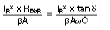…(4)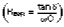for (f=120 Hz)
where tan d and C are measured at 120Hz and w represents 2Pif

From Equation (4) the temperature rise is proportional to the internal resistance (RESR) and is inversely proportional to the heat transfer constant (b) and the surface area of the can. To increase the allowable ripple current, capacitors should have a lower internal resistance (RESR), greater surface area (A) and a higher heat transfer constant (b).

Finally, the relationship between temperature and life approximately meets the following equation: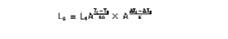Where:
L1 = Significant life at temperature T1
L2 = Significant life at temperature T2
T1 = Maximum rated operating temperature
T2 = Ambient temperature
A = Acceleration coefficient due to ambient temperature
DeltaT1 = Allowed change in core temperature due to ripple current at rated temperature
DeltaT2 = Actual change in core temperature due to ripple current at operating conditions
K = Acceleration coefficient due to applied ripple current

When T2 is less than T1, it is commonly observed that:

A ~= 2 (This acceleration coefficient varies slightly with product series.)

The acceleration coefficient K varies with the change in temperature due to ripple current and the product series.

K ~= 5 ~ 10

For example, with A = 2, K = 5 (typical), T1 = 105°C, T2 = 65°C, DT1 = 5°C and DT2 = 20°C in Equation (1) causes the life to increase by a factor of 2. The lower the operating temperature, the longer the expected life. To achieve still longer life, products having higher maximum allowable operating temperature are recommended.

The ripple current which flows through the capacitor consists of the charging current and the discharging current. When the discharging current flows through the capacitor, the current flows to the anode electrode from the cathode electrode so that, in principle, an oxide layer could form on the cathode electrode. However, in practice this is prevented by suitable capacitor design and selection of cathode foil. Figure 16 shows some examples of how ripple current affects the increase of ESR during a life test.

Figure 16.ESR Change on Life Test with Ripple Current at 85°C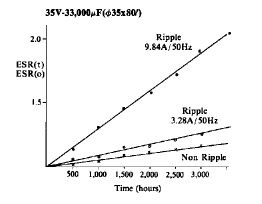Maximum allowable ripple currents (RMS) are normally specified at 120 Hz (sine wave). Since internal resistance (RESR) is frequency dependent, maximum allowable ripple current varies with frequency. (See Table 3.)

Table 3. Ripple Current Multiplier for Frequency
(Typical Values for WV <= 100)

Nominal Capacitance (µF) Frequency (Hz)
50/60 100/120 300 1K 10K 50K
4.7 or below 0.65 1.00 1.35 1.75 2.30 2.50
10 to 47 0.75 1.00 1.25 1.50 1.75 1.80
100 to 1,000 0.80 1.00 1.15 1.30 1.40 1.50

Excessive ripple currents will reduce the life of capac-itors. When designing high ripple current circuits, the customer should select capacitors specially designed for higher ripple current duty. (See Figure 17.)

When applying AC + DC voltage, the peak value of the DC plus superimposed AC voltage should not exceed the rated voltage, and the bottom value should not fall below -1V.

Figure 17. Ripple Current Duty at 85°C